output.to from Sideway
Draft for Information Only

# Content

```Second Moment of Composite Area   Second Moment of Circular Area Example 1   Second Moment of Circular Area Example 2   Negative Area of a composite area   Radius of gyration of composite area```

# Second Moment of Composite Area

By definition, second moment of an area about an axis is equal to the summation of the products of the square of the distance between the elemental area and the reference axis, and the elemental area over an area. If these elemental areas can be grouped into known component areas A1, A2, A3, ...., the second moment I of the composite area A with respect to an axis can be obtained by the summation of the second moments, I1, I2, I3, .... of these component areas A1, A2, A3, ...., about the same reference axis respectively.  Imply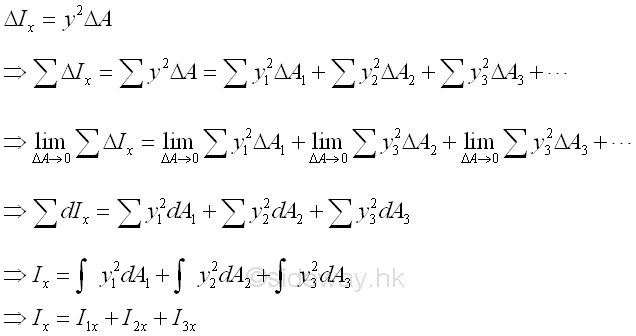The component area of a composite area is represented by a positive area while a hollow area is represented by a negative area.

### Second Moment of Circular Area Example 1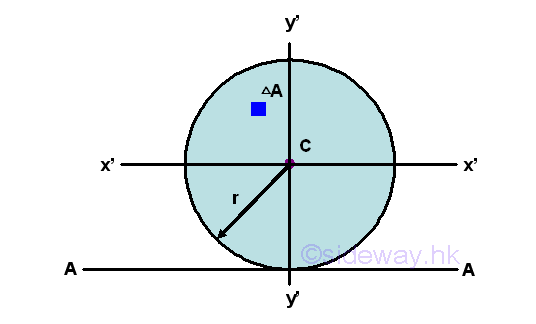Since a circular area can be considered as two semi-circular area or four quarter-circular area, the second moment of a circular area about the centroidal axis x' can be expressed in terms of the second moments of semi-circular areas or quarter-circular areas. As for two semi-circular areas,Because of the square of the distance from the reference axis x', the second moments of the two semi-circular areas about axis x' are the same on two opposite sides of the reference axis. Imply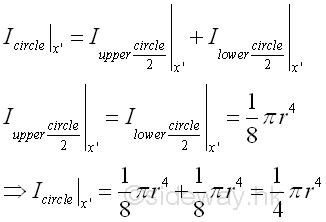As four quarter-circular areas,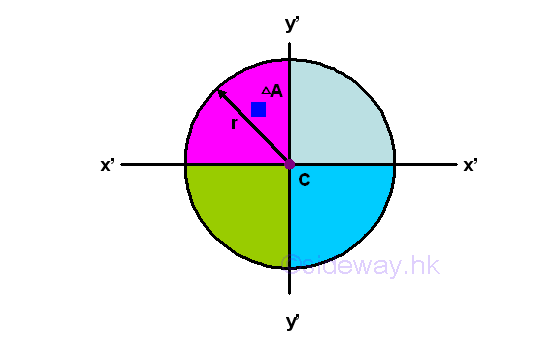Because of the square of the distance from the reference axis y', the second moments of the four quarter-circular areas about axis y' are the same on two opposite sides of the reference axis. Imply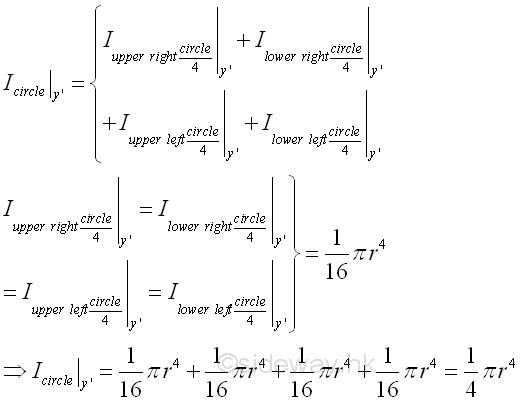Besides, the polar moment of an area can also be obtained similarly. Because of the square of the distance from the reference pole C, the second moments of the four quarter-circular areas about the pole C are the same. Imply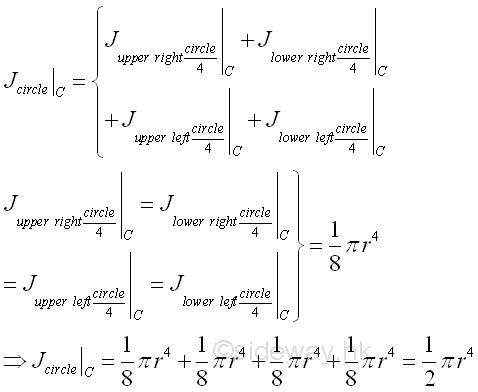### Second Moment of Circular Area Example 2In example 1, the reference axis x' of the circular area is the same axis used in defining the second moment of the semi-circular areas and the quarter-circular areas, the second moments of the component areas can be added directly. But if the reference axis is changed to axis A, each second moment of a component area should be transfered to the desired axis A by parall-axis theorem or by calculation before adding.  As for two semi-circular areas,Since the two second moments of the two semi-circular areas are about the axis x', the two second moments of the two semi-circular areas should be transfered to the axis A before adding accordingly. Imply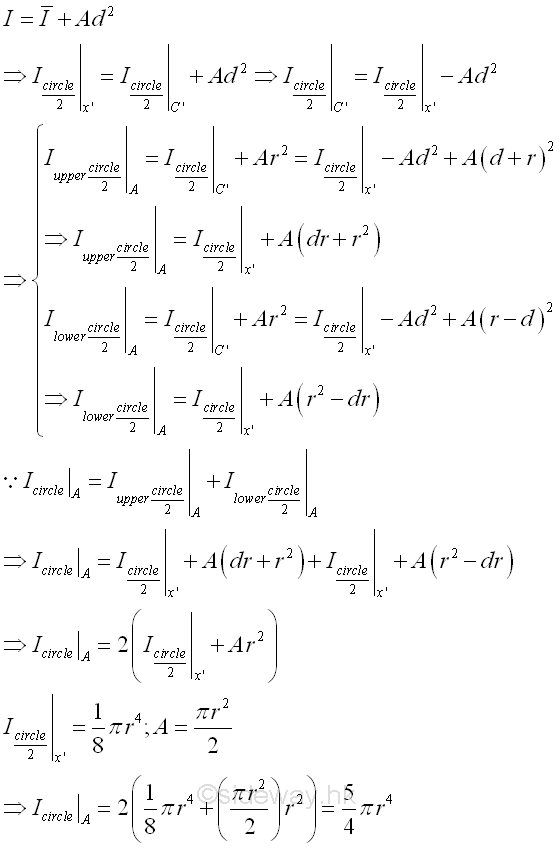### Negative Area of a composite area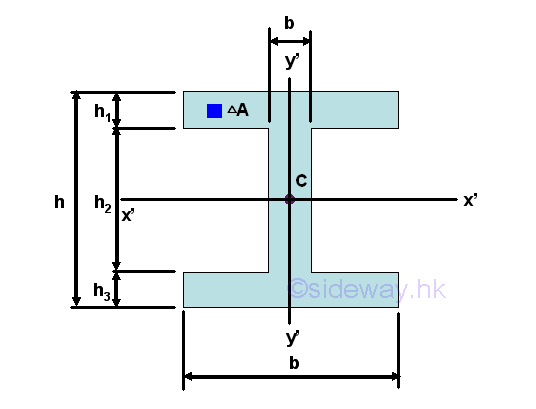Sometimes, an empty area in a composite area can also be considered in calculating the second moment of a composite area by changing the sign of the empty area to negative before adding. And therefore the empty component area of a composite area is also called negative area. For example, the second moment of an I-beam area starting with all positive components. ImplyTherefore, the second moment of an I-beam area starting with positive and negative components. Imply### Radius of gyration of composite area

Unlike the polar radius of gyration, kO which can be obtained using the two rectangular radius of gyration, kx and ky only, the radius of gyration of a composite area can not be obtained using the radii of gyration of all component areas of a composite area only. The radius of gyration of a composite area can be determined by the second moment of the composite area and the area of the composite area directly or making use of the radii of gyration of all component areas of a composite area together with the areas of the component areas of a composite area. Imply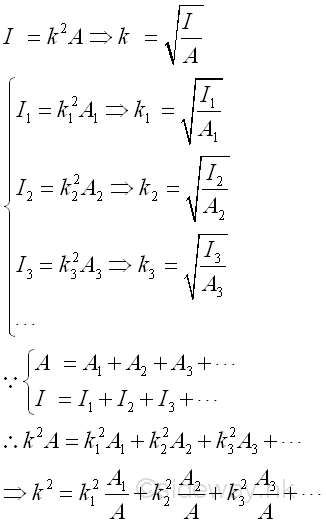ID: 121000009 Last Updated: 18/10/2012 Revision: 0 Ref:References

1. F.P. Beer; E.R. Johnston,Jr.; E.R. Eisenberg, 2004, Vector Mechanics for Engineers: Statics
2. I.C. Jong; B.G. rogers, 1991, Engineering Mechanics: Statics and DynamicsHome 5

Management

HBR 3

Information

Recreation

Culture

Chinese 1097

English 337

Computer

Hardware 151

Software

Application 202

Latex 10

Manim 159

Numeric 19

Programming

Web 285

Unicode 494

HTML 65

CSS 59

ASP.NET 194

OS 391

Python 36

Knowledge

Mathematics

Algebra 29

Geometry 18

Calculus 67

Engineering

Mechanical

Rigid Bodies

Statics 92

Dynamics 37

Control

Natural Sciences

Electric 27